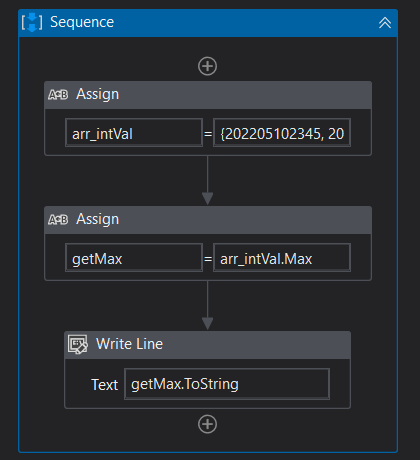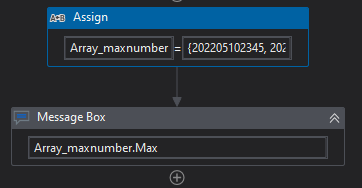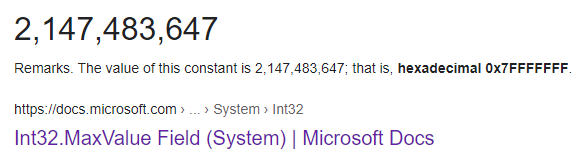# Get Largest number out of Array of Strings

Hi,
I face a scenario where I need the Highest number out of an Array of Strings.
Array of Strings = {202205102345, 202205112210, 202205123375}
I used the function,
GetMax = ArrayofStrings.Max(function(e) convert.toint32(e))

Where Getmax is Int32.
I get only the last 2 digits of the highest number for GetMax. In the above case, getmax =75
But I need the entire Value (ie. 202205123375)
Can someone help.

1 Like

Kindly try the below,

``````strArr_Num = {"202205102345","202205112210","202205123375"}

strArr_Num.Max(Function(num) num)
``````

Ref - nmnithinkrishna_MaxNumFromStrArray.zip (2.0 KB)

Hope this helps.

Thanks
#nK

1 Liketry arr_int64.Max

Hi,

Hope the following helps you.

``````arrStr = {"202205102345","202205112210","202205123375"}
``````

Then

``````arrStr.OrderBy(Function(s) CInt(Strings.Right(s,2))).Last()
``````

The above evaluates last 2 digits and return whole number string which has the max 2 digits : “202205123375”

Regards,

Maybe try Converting the `GetMax` variable type to `Double` and Check or `BigInteger`

Try thisVariable type for Array_maxnumber is System.Int64[]

I hope this may work for you …
If you find it useful mark it as a solution and close the thread.

Regards,
Gulshiyaa

The max value for Int32 is 2,147,483,647. Your value of 202,205,123,375 is too big for the variable to hold. As suggested by the others, use Int64 instead.

Declare `GetMax` as Int64 and then assign it as below:
`GetMax = ArrayofStrings.Max(Function(e) Convert.ToInt64(e))`